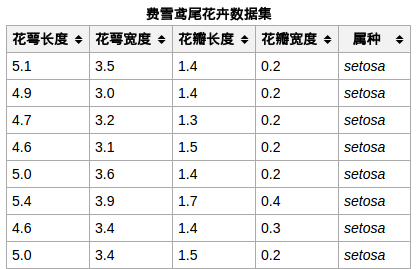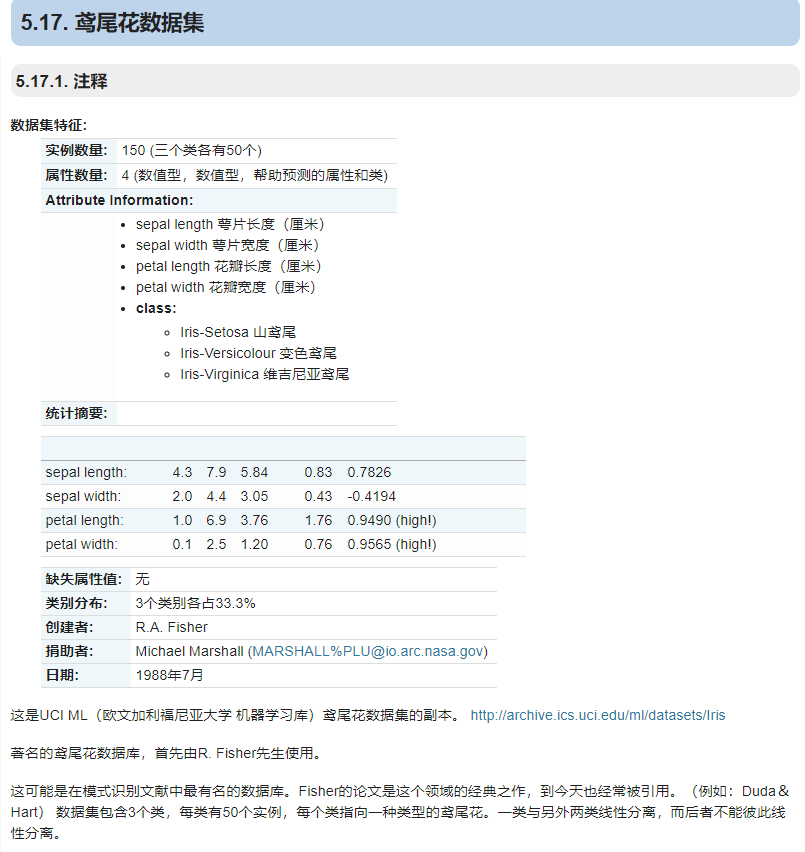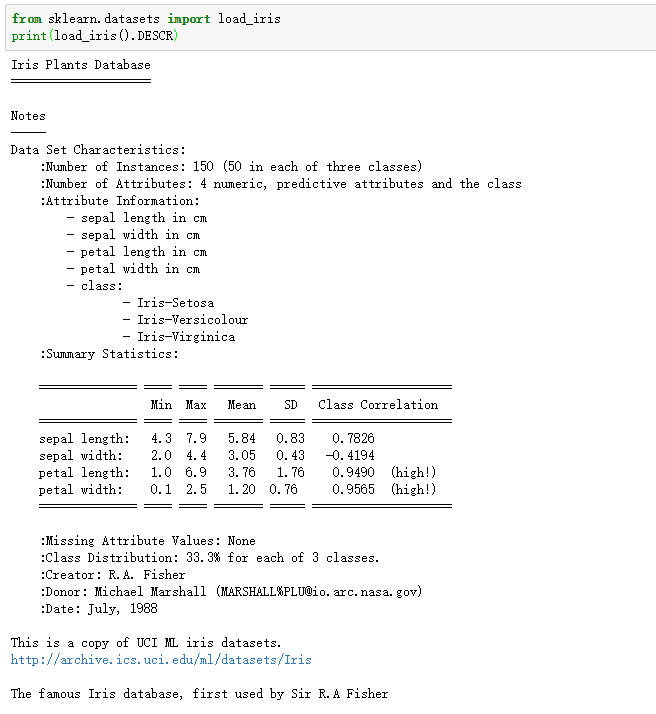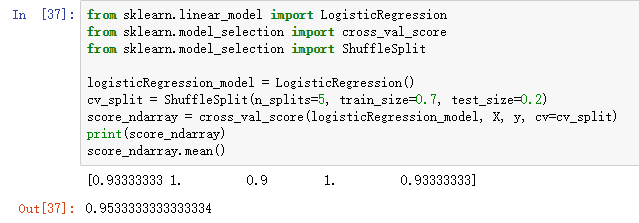#0
0
01. 云栖社区>
2. 博客>
3. 正文

## 基于LogisticRegression的鸢尾花分类

LogisticRegression中文叫做逻辑回归，是一种基础、常用的分类方法。

2018年8月24日笔记

## 1.数据集

Iris（鸢尾花）数据集是多重变量分析的数据集。Iris.png

1.完整的样本数据Iris.csv文件下载链接: https://pan.baidu.com/s/16N0ivGWFrmc73ustPLWPZA 密码: ugun
2.数据集链接：https://gist.github.com/curran/a08a1080b88344b0c8a7

## 2.观察数据image.png

``````from sklearn.datasets import load_iris
``````

['DESCR', 'data', 'feature_names', 'target', 'target_names']

``````from sklearn.datasets import load_iris
``````image.png

``````from sklearn.datasets import load_iris
``````

## 3.逻辑回归模型

``````from sklearn.linear_model import LogisticRegression
from sklearn.model_selection import cross_val_score
from sklearn.model_selection import ShuffleSplit

logisticRegression_model = LogisticRegression()
cv_split = ShuffleSplit(n_splits=5, train_size=0.7, test_size=0.2)
score_ndarray = cross_val_score(logisticRegression_model, X, y, cv=cv_split)
print(score_ndarray)
score_ndarray.mean()
``````+ 关注